# Power Factor Correction: What is it? (Formula, Circuit And Capacitor Banks)

Contents

## What is Power Factor Correction?

Power factor correction (also known as PFC or Power Factor Improvement) is defined as a technique used to improve the power factor of AC circuits by reducing the reactive power present in the circuit. Power factor correction techniques aim to increase the efficiency of the circuit and reduce the current drawn by the load.

Generally, capacitors and synchronous motors are used in circuits to reduce the inductive elements (and hence the reactive power). These techniques are not used to increase the amount of true power, only to decrease the apparent power.

In other words, it reduces the phase shift between voltage and current. So, it tries to keep the power factor near to the unity. The most economical value of the power factor is between 0.9 to 0.95.

Now the question arises, why the economical value of the power factor is 0.95 instead of the unity power factor? Is there any disadvantage of the unity power factor?

NO. There is not a single disadvantage of the unity power factor. But it is difficult and costly to install unity PFC equipment.

Therefore, utility and power supply companies try to make a power factor in a range of 0.9 to 0.95 to make an economic system. And this range is good enough for a power system.

If the AC circuit has a high inductive load, the power factor may lie below 0.8. And it draws more current from the source.

The power factor correction equipment reduces inductive elements and current drawn from the source. It results in an efficient system and prevents the loss of electrical energy.

## Why Power Factor Correction is Needed?

In DC circuits, the power dissipated by a load is simply calculated by multiplying voltage and current. And the current is proportional to an applied voltage. Therefore, the power dissipation by the resistive load is linear.

In AC circuits, voltage and current are sinusoidal waves. Hence, the magnitude and direction change continuously. At the particular instant of time, the power dissipated is a multiplication of voltage and current at that instant.

If an AC circuit having inductive loads like; winding, chowk coils, solenoid, transformer; the current is out of phase with the voltage. In this condition, actual power dissipated is less than the product of voltage and current.

Due to non-linear elements in AC circuits, it contains both resistance and reactance. Therefore, in this condition, the phase difference of current and voltage is important while calculating the power.

For pure resistive load, the voltage and currents are in phase. But for inductive load, the current lags behind the voltage. And it creates inductive reactance.

In this condition, the power factor correction is most needed to reduce the effect of the inductive element and improve the power factor to increase the efficiency of the system.

## Power Factor Correction Formula

Consider an inductive load is connected with the system and operates at power factor cosф1. To improve the power factor, we need to connect power factor correction equipment in parallel with the load.

The circuit diagram of this arrangement is shown below figure.

The capacitor supplies leading reactive component and reduce the effect of lagging reactive component. Before connecting capacitor, the load current is IL.

The capacitor takes IC current that leads voltage by 90˚. And the resultant current of the system is Ir. Angle between voltage V and IR is decreased compared to angle between V and IL. Therefore, the power factor cosф2 is improved.

From the above phaser diagram, the lagging component of the system is reduced. Hence, to change the power factor from ф1 to ф2, the load current is reduced by IRsinф2.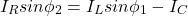Capacitance of capacitor to improve power factor is;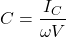## Power Factor Correction Circuit

Power factor correction techniques mainly used capacitor or capacitor bank and synchronous condenser. According to the equipment used to correct the power factor, there are three methods;

• Capacitor Bank
• Synchronous Condenser

### Power Factor Correction using Capacitor Bank

The capacitor or capacitor bank can be connected as fixed or variable value capacitance. It is connected to an induction motor, distribution panel, or main supply.

The fixed value capacitor is connected continuously with the system. A variable value capacitance varies the amount of KVAR according to the requirement of the system.

For power factor correction, the capacitor bank is used to connect with the load. If the load is a three-phase load, the capacitor bank can be connected as a star and delta connection.

#### Delta Connected Capacitor Bank

The below circuit diagram shows delta connected capacitor bank with a three-phase load.

Let’s find the equation of capacitor per phase when it is connected in delta connection. In delta connection, the phase voltage (VP) and line voltage (VL) are equal.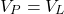The capacitance per phase (C) is given as;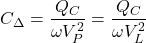#### Star Connected Capacitor Bank

The below circuit diagram shows star connected capacitor bank with a three-phase load.

In star connection, the relation between phase voltage (VP) and line voltage (VL) is;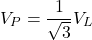The capacitance per phase (CY) is given as;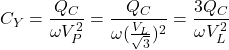From the above equations;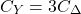It means that the capacitance requires in the star connection is three times the capacitance requires in the delta connection. And also, the operating phase voltage is 1/√3 times line voltage.

So, the delta-connected capacitor bank is a good design and that is the reason, in a three-phase connection, the delta-connected capacitor bank is used more in the network.

### Power Factor Correction using Synchronous Condenser

When a synchronous motor is overexcited, it takes the leading current and behaves as a capacitor. An over-excited synchronous motor running on no-load condition is known as the synchronous condenser.

When this type of machine is connected in parallel with supply, it takes a leading current. And improves the power factor of the system. The connection diagram of the synchronous condenser with supply is as shown in the below figure.

When the load has a reactive component, it draws a lagging current from the system. To neutralize the current, this device is used to take a leading current.

Before the synchronous condenser is connected, the current drawn by the load is IL and the power factor is фL.

When the synchronous condenser is connected, it takes current Im. In this condition, the resultant current is I and the power factor is фm.

From the phasor diagram, we can compare both power factor angles (фL and фm). And фm is less than фL. Therefore, the cosфm is greater than cosфL.

This type of power factor improvement method is used at bulk supply stations due to the below advantages.

• The magnitude of current drawn by the motor is changed by varying the field excitation.
• It is easy to remove faults that occur in the system.
• The thermal stability of motor winding is high. Hence, it is a reliable system for short circuit currents.

Induction motor draws reactive current due to excitation current. If another source is used to provide excitation current, the stator winding free from the excitation current. And the power factor of a motor can be improved.

This arrangement can be done by using the phase advancer. The phase advancer is a simple AC exciter mounted on the same shaft of the motor and connected with the rotor circuit of the motor.

It provides excitation current to the rotor circuit at slip frequency. If you provide more exciter current than required, the induction motor can be operated on leading power factor.

The only disadvantage of phase advancer is that it is not economical for small size motor, especially below 200 HP.

## Active Power Factor Correction

Active power factor correction provides more efficient power factor control. Generally, it is used in power supply design for more than 100W.

This type of power factor correction circuit consists of high-frequency switching elements like a diode, SCR (power electronics switches). These elements are active elements. Therefore, this method is named as Active power factor correction method.

In passive power factor correction, the reactive elements like capacitor and inductor used in the circuit are uncontrolled. As passive power factor correction circuit not using any control unit and switching elements.

Due to the high switching elements and control unit used in the circuit, the cost and complexity of the circuit are increased compared to the passive power factor correction circuit.

The below circuit diagram shows the basic elements of an active power factor correction circuit.

To control the circuit parameters, a control unit is used in the circuit. It measures the input voltage and current. And it adjusts switching time and duty cycle in phase voltage and current.

The inductor L is controlled by solid-state switch Q. The control unit is used to control (ON and OFF) the solid-state switch Q.

When the switch is ON, the inductor current increases by ∆I+. The voltage across the inductor reverses polarity and release to accumulate energy via diode D1 to the load.

When the switch is OFF, the inductor current decrease by ∆I. The total change during one cycle is ∆I = ∆I+ – ∆I. The ON and OFF time of the switch is controlled by the control unit by changing the duty cycle.

By proper selection of duty cycle, we can get the desired shape of the current to the load.

## How to Size Power Factor Correction?

To size the power factor correction, we need to calculate the requirement of reactive power (KVAR). And we connect that size of capacitance with the system to meet the reactive power demand.

There are two ways to find the requirement of KVAR.

• Table Multiplier Method
• Calculation Method

As the name suggests, in the table multiplier method, we can directly find a multiplier constant from a table. We can directly find the required KVAR by multiplying the constant with the input power.

In the calculation method, we need to calculate the multiplier as shown below example.

Example:

A 10-kW induction motor has a power factor of 0.71 lagging. If we need to run this motor at a power factor of 0.92, what will be the size of the capacitor?

Input Power = 10kW
Actual Power Factor (cos фA) = 0.71
Required Power Factor (cos фR) = 0.92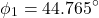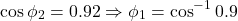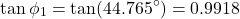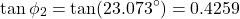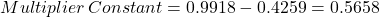Required KVAR = Input Power x Multiplier Constant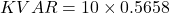Therefore, 5.658 KVAR reactive power is required to improve the power factor from 0.71 to 0.92. And capacitor connected with the system has a capacitance of 5.658 KVAR.

## Applications of Power Factor Correction

In a power system network, the power factor plays a most important role in the quality and management of the system. It determines the efficiency of the power supply.

• Without power factor correction, the load draws a high magnitude current from the source. It increases the losses and cost of electric energy. PFC equipment tries to make current and voltage waveform in phase. This will increase the efficiency of the system.
• In the transmission network, a high-power factor is necessary. Due to the high-power factor, the losses of the transmission line are decreased and improves the voltage regulation.
• The induction motor is widely used equipment in industries. To avoid overheating and improve the efficiency of a motor, the capacitors are used to mitigate the effect of reactive power.
• PFC equipment reduces the heat generation in cables, switchgear, alternator, transformers, etc.
• Due to the high efficiency of the network, we need to generate less energy. Which reduce carbon emission into the atmosphere.
• Voltage drop considerably reduces by using PFC equipment with the system.

Want To Learn Faster? 🎓
Get electrical articles delivered to your inbox every week.
No credit card required—it’s 100% free.